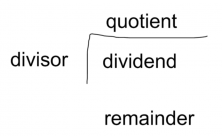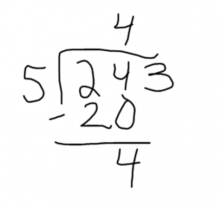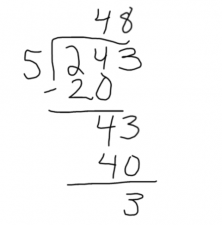Smartick is an advanced online program that teaches kids math and coding in only 15 min. a day

May31

# Learn to Divide by One Digit with an Example

In this post, we are going to learn to divide by one digit.  For that, we must first know what to call each part of a division problem:

• The dividend is the number that is going to be divided.
• The divisor is the number that the dividend is divided by.
• The quotient is the result of the division problem.
• The remainder is the leftover quantity.### For example

We want to distribute 243 caramels among 5 friends. The dividend will be 243 because it is the amount that we want to divide, the divisor is 5 because it is the number by which we want to divide (share) the 243 caramels. The quotient will be the number caramels that each child receives and the remainder are the caramels that are left over.

We are going to see the steps in order to calculate the quotient and remainder:

1. We take the first digit of the dividend. If that digit is less than the divisor, then we have to take another digit of the dividend.

In our example, the first digit of the dividend is 2, but since it is less than the divisor, which is 5, we have to take another digit: 24

1. Look for a number that when multiplied by the divisor will give us the number formed by these digits of the dividend. If that does not exist, we look for the greatest number that can be multiplied by the divisor to produce a result that is less than the number formed by the digits we have taken from the dividend. The result of the multiplication is subtracted from the dividend.

We have to divide 24 by 5. Look for a number which multiplies by 5 to give us 24. As there is no exact fit, we notice that 5×5=25 is greater than 24, so we take the next smallest number: 4 x 5 = 20. In this case, 20 is the greatest multiple of 5 that is less than 24. Therefore, we write 4 in the quotient and subtract the 20 from 24, and 20 – 24 = 4.1. Bring down the next digit of the dividend and return to step 2.

We drop the next digit, which is the 3. With the 4 that we already have and the 3 that we just dropped, we get 43.

Now we divide 43 by 5. Look for a number which multiples by 5 to give us 43. As there is no exact fit, we look for the greatest number of 8’s that is less than 43. In this case, 5 x 8 = 40. We write the 8 in the quotient and the 40 beneath the 43 to subtract: 43 – 40 = 3.As there are no more digits in the dividend, we are finished with the division problem.

Therefore, each child receives 48 caramels and 3 caramels remain left over.

If you want to see a more detailed explanation of how to divide with more examples, visit our posts: